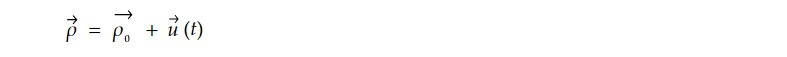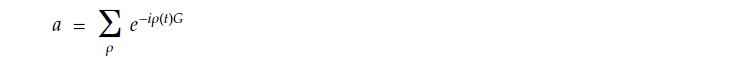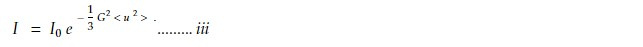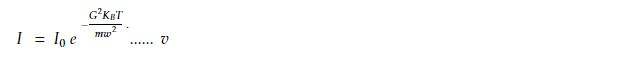# Effect of temperature on the intensity of Bragg's reflected beam.

The intensity of Bragg's reflected beam in X-ray or neutron diffraction experiments is influenced by temperature through the Debye-Waller correction. As the temperature increases, the atomic vibrations or thermal motion of the crystal lattice become more significant, leading to a reduction in the measured intensity but the angular width of reflected line doesn't change.

Let position of the atom normally ρo contain the term u(t) fluctuating in time. i.ewhere ρ(t) is position of atom at time t

The radiation amplitude scattered by a crystal is given byG is reciprocal latticeThe series expansion of exponential term is given byas vector u is a random thermal displacement, uncorrected with direction of vector G.Thus equation 1 can be written asnow the scattered intensity which is the square of amplitude is given by

I ∝ a²where Io is the scattered intensity of rigid lattice. The exponential factor is the Debye Waller factor and < u²> mean square displacement of the atom.

For classical harmonic oscillator (3-D) average potential energy is

< U> = 1/2 mw² <u ²> = 3/2 K_BT

<u ²> = 3K_BT / mw² .....iv

Using iv in iii, we get< u² > = 0 at T =0

But for Q harmonic oscillator, < u² > doesn't vanish at T = 0. there is zero point energy. The energy of harmonic oscillator in ground state is 3/2 ℏ w

< u² > = 1/2 m w² < u² > = 3/4 ℏ w

=> < u² > = 3 ℏ / 2mw ......vi

From iii and vi,at T = 0k

At higher temperatures, the atomic vibrations become more pronounced, resulting in larger values of the Debye-Waller parameter. Consequently, the Debye-Waller factor becomes smaller, causing a decrease in the measured intensity of the Bragg's reflected beam.

Therefore, as the temperature increases, the intensity of the Bragg's reflected beam tends to decrease due to the influence of thermal motion and the associated Debye-Waller correction. This effect needs to be considered and corrected for when analysing diffraction data to obtain accurate structural information from a crystal..

This note is a part of the Physics Repository.